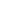# Intelligence Bureau JIO Electrical Skill Test important Questions

###### IB JIO Skill Test & Interview Preparation

Electrical Important Questions & Topic For IB Interview

Skill test is part of interview process for the selection post of Junior Intelligence Officer Post by Intelligence Bureau in Ministry of Home Affair. The skill test will be based upon the practical knowledge of the technical subject commensurate with the job profile. The skill test for JIO post will be technical in nature. The technical practical based questions will be asked from the respective branch or field of knowledge of the candidate.

## Electrical Important Questions & Topic For IB Interview

• What personal protective equipment PPE should you wear when working in an electrical lab and why is it important?

• Explain the proper procedure for inspecting electrical equipment before use.

• Describe the emergency procedures to follow in case of an electrical accident.

• How can you verify that an electrical circuit is de-energized before starting work on it?

• Calculate the resistance of a resistor with color bands of brown, black, and red?

• Draw the schematic diagram of a simple series circuit with a resistor and an LED?

• Explain the difference between a breadboard and a PCB for prototyping electrical circuits?

• Calculate the total resistance in a parallel circuit with three resistors?

• Voltage and Current Measurement Using a multimeter, measure the voltage across a 9V battery?

• Measure the current through a resistor using an ammeter?

• Explain the concept of voltage division and how to calculate voltages in a series circuit?

• Describe the function of an oscilloscope and how to measure the frequency of an AC signal?

• Identify the symbol and function of a diode?

• List three common applications of capacitors in electrical circuits?

• Explain the operation of an NPN transistor and its use as a switch?

• How does a relay work, and provide an example of its use in a circuit?

• Design a simple logic gate circuit using AND, OR, and NOT gates?

• Convert a decimal number to its binary representation?

• Explain the purpose of a microcontroller in embedded system?

• Program a microcontroller to flash an LED in a specific pattern?

• Describe the working principle of a DC motor and its applications?

• Calculate the speed of a three-phase induction motor given its synchronous speed and slip?

• Explain the construction and operation of a transformer?

• Perform a polarity test on a single-phase transformer?

• Calculate the real power, apparent power, and power factor of a load given voltage and current values?

• Describe the function of a circuit breaker in protecting electrical circuits?

• Calculate the line and phase currents in a balanced three-phase system?

• Identify common faults in electrical circuits, such as open circuits, short circuits, and loose connections?

• Explain the procedure for using a megohmmeter to test insulation resistance?

• Troubleshoot a circuit where an LED isn't lighting up as expected?

• Describe how to identify a blown fuse and replace it safely?Request
Call back